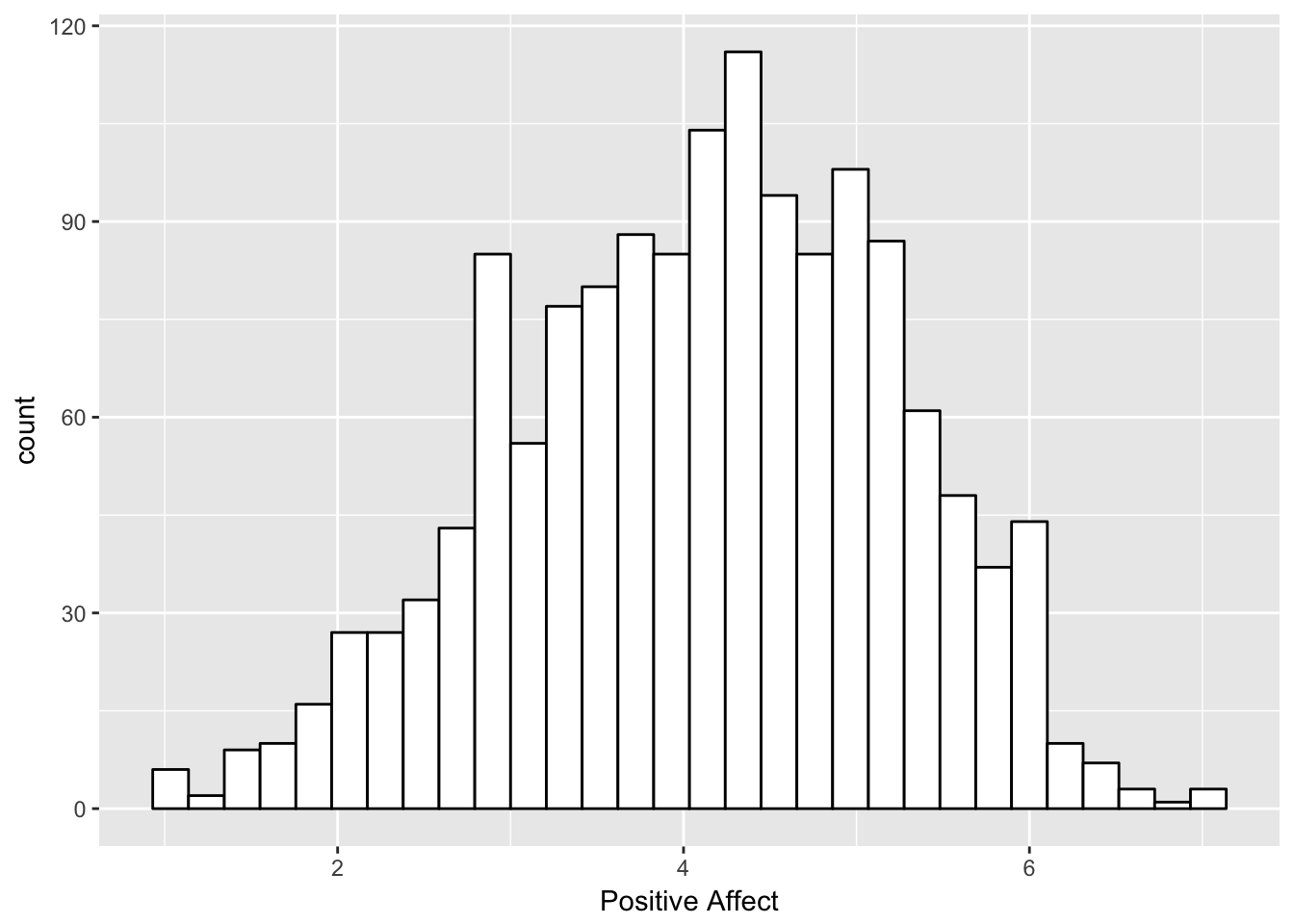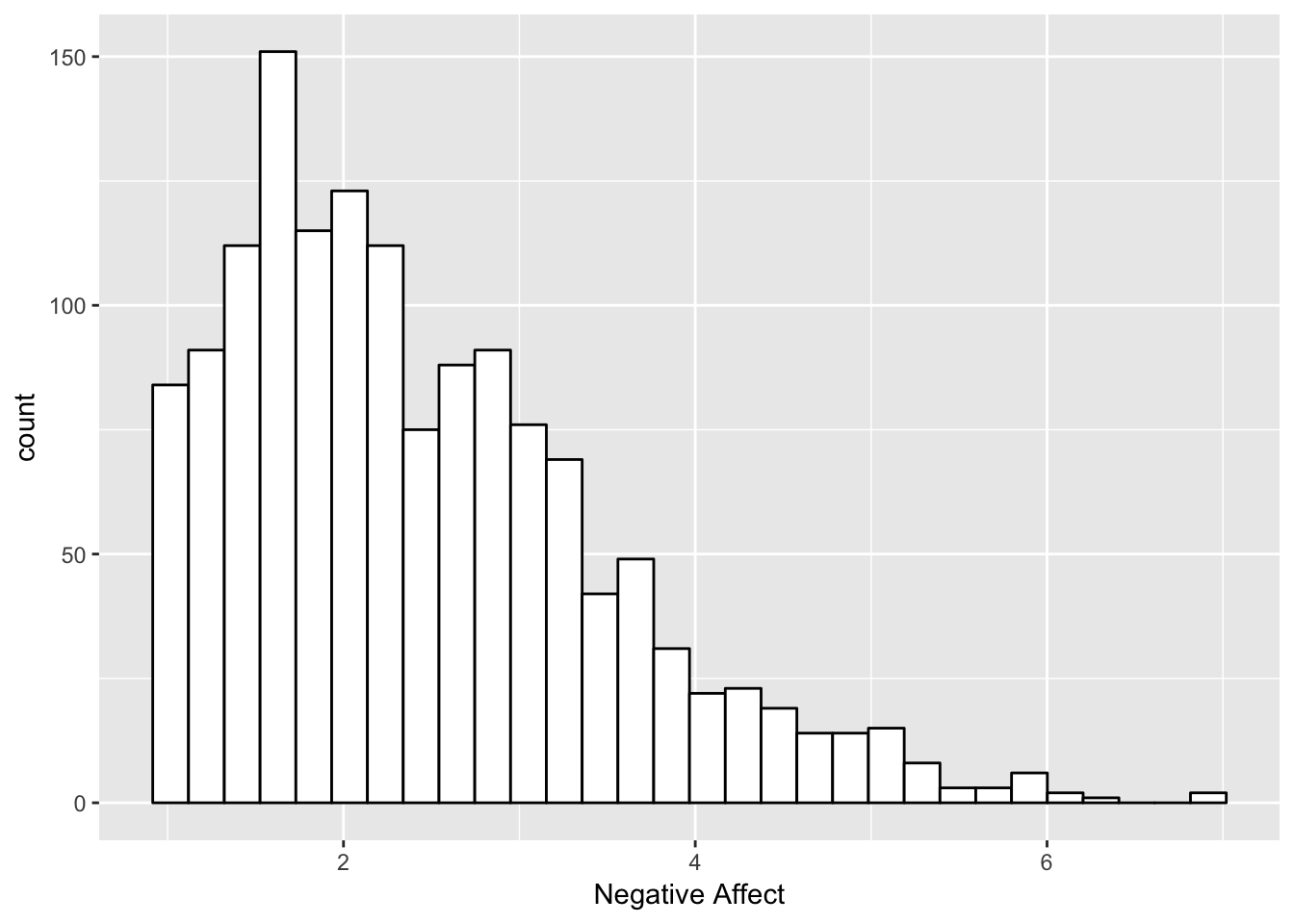## Overview

This tutorial continues our walk through calculation of intraindividual variability metrics. Specifically, this tutorial demonstrates calculation of intraindividual summary statistics using equations that get translated into functions. As with other measures, the derived metrics are useful for articulating a variety of dynamic characteristics from experience sampling data, and as demonstrated at the end of this session, examining how those characteristics are related to other individual differences variables.

## Outline

In this session we cover …

A. Review description of data and calculation of basic intaindividual variability (IIV) metrics.
B. Calculating some additional IIV metrics (MSSD, PAC) based on their formulas.
C. Examining how the IIV metrics are related to other individual differences variables using regressions.

``````library(psych)
library(ggplot2)
library(data.table)
library(entropy)
library(plyr)
library(zoo)``````

#### Prelim - Reading in Repeated Measures Data

Note that we are working from a long file. For your own data, there may be some steps to get to this point.

``````#Setting the working directory
setwd("~/Desktop/Fall_2017")  #Person 1 Computer
#setwd("~/Desktop/Fall_2017")  #Person 2 Computer

#set filepath for data file
filepath <- "https://quantdev.ssri.psu.edu/sites/qdev/files/AMIBbrief_raw_daily1.csv"
#read in the .csv file using the url() function

#Little bit of clean-up
var.names.daily <- tolower(colnames(daily))
colnames(daily)<-var.names.daily``````

Everything we do today uses a long file.

## A: Looking at distributions and within-person time-series

Lets look at some descriptions of today’s variables.

``````#getting a list of the variable names
names(daily)``````
``````##   "id"      "day"     "date"    "slphrs"  "weath"   "lteq"    "pss"
##   "se"      "swls"    "evalday" "posaff"  "negaff"  "temp"    "hum"
##  "wind"    "bar"     "prec"``````
``````#sample descriptives
describe(daily\$posaff)``````
``````##    vars    n mean  sd median trimmed  mad min max range  skew kurtosis
## X1    1 1441 4.12 1.1    4.2    4.15 1.19   1   7     6 -0.25    -0.33
##      se
## X1 0.03``````
``describe(daily\$negaff)``
``````##    vars    n mean   sd median trimmed  mad min max range skew kurtosis
## X1    1 1441 2.45 1.04    2.2    2.34 1.04   1 6.9   5.9 0.96     0.77
##      se
## X1 0.03``````
``````#histograms
ggplot(data=daily, aes(x=posaff)) +
geom_histogram(fill="white", color="black") +
labs(x = "Positive Affect")``````
``## `stat_bin()` using `bins = 30`. Pick better value with `binwidth`.``
``## Warning: Removed 17 rows containing non-finite values (stat_bin).````````ggplot(data=daily, aes(x=negaff)) +
geom_histogram(fill="white", color="black") +
labs(x = "Negative Affect")``````
``## `stat_bin()` using `bins = 30`. Pick better value with `binwidth`.``
``## Warning: Removed 17 rows containing non-finite values (stat_bin).``We can see the differences in the sample-level distributions (all persons AND all occasions) between the two variables.

And lets look at the longitudinal (“spaghetti”) plots …
1. Positive Affect

``````#ggplot version .. see also http://ggplot.yhathq.com/docs/index.html
ggplot(data = daily[which(daily\$id >=527),], aes(x = day, y = posaff, group = id, color=factor(id))) +
geom_point() +
geom_line(data=daily[which(daily\$id >=527 & daily\$posaff !="NA"),]) +
xlab("Day") +
ylab("Positive Affect") + #ylim(1,7) +
scale_x_continuous(breaks=seq(0,7,by=1)) ``````